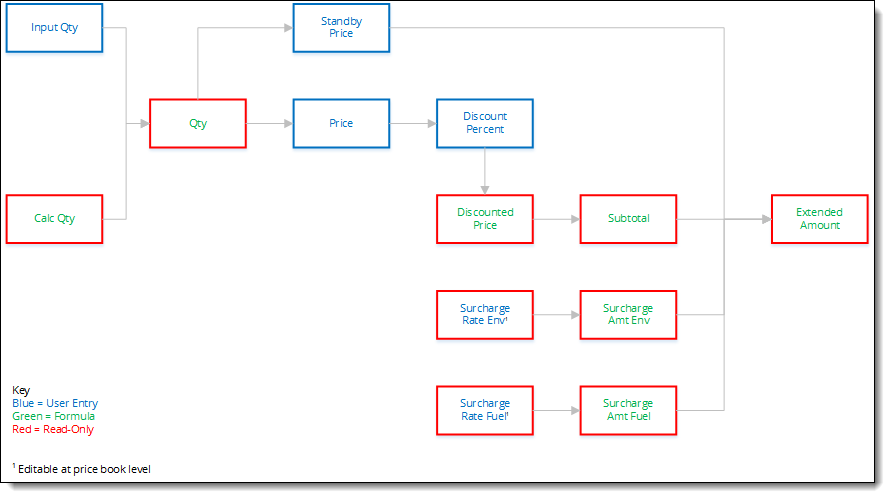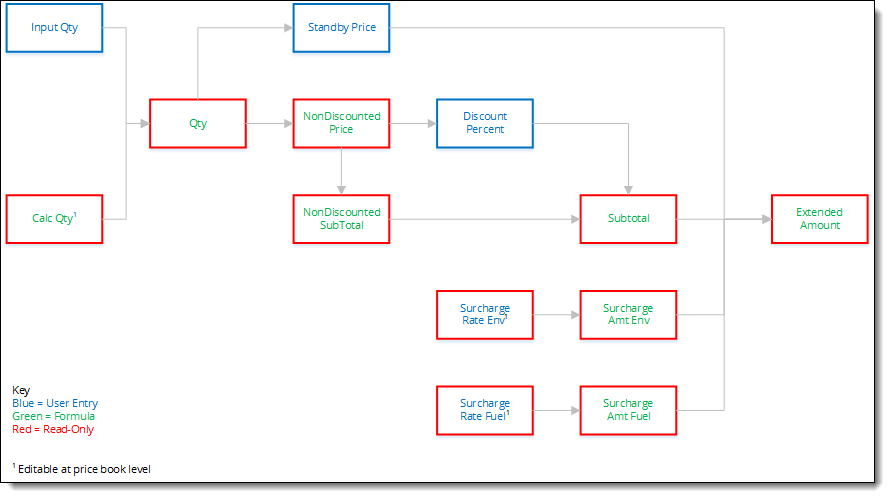# Extended Amount Field

## Basics

The Extended Amount field is a formula field on the Ticket Item object that displays the amount charged for a ticket item.

Here’s the default formula for the Extended Amount field:• User inputted qty and the read only qty fields are used to determine the quantity

• User entered standby price

• User entered price and user entered discount are used to calculate a discounted price and then added to a subtotal

• Inputted or formulaic surcharges are calculated

• Inputted or formulaic fuel surcharges are calculated

## Stage Pricing

The formula for the Extended Amount field changes to the following if you set up the Stage Pricing feature:• User inputted qty and the read only qty fields are used to determine the quantity

• User entered standby pricing

• Subtotal of items is calculated:

• Non-discountable prices are calculated into a subtotal

• User entered discounts are applied to discountable quantities to calculate a subtotal on discountable items

• User entered or formulaic surcharges are calculated

• User entered or formulaic fuel surcharges are calculated